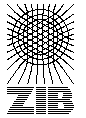# Testset P6E

These instances are random generated sparse graphs with euclidian weights. The were introduced in CGR92 .

Name   |V|    |E|    |T|    DC    Opt
p619100 180 Ls 7485
p620100 180 Ps 8746
p621100 180 Ls 8688
p622100 180 10 Ps 15972
p623100 180 10 Ps 19496
p624100 180 20 Ls 20246
p625100 180 20 Ps 23078
p626100 180 20 Ls 22346
p627100 180 50 Ps 40647
p628100 180 50 Ls 40008
p629100 180 50 Ls 43287
p630200 370 10 Ps 26125
p631200 370 20 Ps 39067
p632200 370 40 Ps 56217
p633200 370 100 Ls 86268

The column DC classifies the difficulty of the instance.

L
Solvable by usage of local preprocessing. Typical examples are the SD-Test, BD-n Tests and FST computations. Neither a global upper nor lower bound needs to be computed.
P
Solvable by polynomial time algorithms, like dual ascent in combination with primal heuristic, a integral LP formulation or advanced preprocessing like reduced cost criteria or the RCR-Test.
NP
No polynomial time algorithm is known. Use of an exponential time enumeration sceme like Branch-and-Bound is neccessary.

The letter after class gives an impression how long it takes to solve the problem using state-of-the-art soft- and hardware. secounds means less than a minute (this includes instances which can be solved in fractions of a second). minutes means less than an hour. hours is less than a day and days is less than a week. weeks mean it takes really a long time to solve this instance. ? means the instance is not solved or the time is not known.

If the number in the Opt column is written in italics the optimum is not known. The number given is the best know upper bound.

Last Update : 2015/02/11 11:57:20 \$ by Thorsten Koch